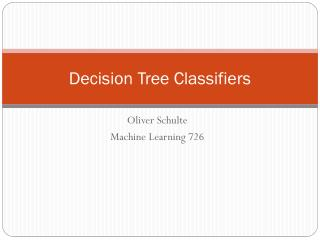DownloadDownload PresentationDecision Tree Classifiers

# Decision Tree Classifiers

Télécharger la présentation## Decision Tree Classifiers

- - - - - - - - - - - - - - - - - - - - - - - - - - - E N D - - - - - - - - - - - - - - - - - - - - - - - - - - -
##### Presentation Transcript

1. Decision Tree Classifiers Oliver Schulte Machine Learning 726

2. Overview

3. Decision Tree • Popular type of classifier. Easy to visualize. • Especially for discrete values, but also for continuous. • Learning: Information Theory.

4. Decision Tree Example

5. Exercise Find a decision tree to represent • A OR B, A AND B, A XOR B. • (A AND B) OR (C AND notD AND E)

6. Decision Tree Learning • Basic Loop: • A := the “best” decision attribute for next node. • For each value of A, create new descendant of node. • Assign training examples to leaf nodes. • If training examples perfect classified, then STOP.Else iterate over new leaf nodes.

7. Entropy

8. Uncertainty and Probability • The more “balanced” a probability distribution, the less information it conveys (e.g., about class label). • How to quantify? • Information Theory: Entropy = Balance. • S is sample, p+ is proportion positive, p- negative. • Entropy(S) = -p+log2(p+) - p-log2(p-)

9. Entropy: General Definition • Important quantity in • coding theory • statistical physics • machine learning

10. Intuition

11. Entropy

12. Coding Theory • Coding theory: Xdiscrete with 8 possible states (“messages”); how many bits to transmit the state of X? • Shannon information theorem: optimal code length assigns p(x) to each “message” X = x. • All states equally likely

13. Another Coding Example

14. Zipf’s Law • General principle: frequent messages get shorter codes. • e.g., abbreviations. • Information Compression.

15. The Kullback-Leibler Divergence Measures information-theoretic “distance” between two distributions p and q. Code length of x in true distribution Code length of x in wrong distribution

16. Information Gain

17. Splitting Criterion • A new attribute value changes the entropy. • Intuitively, want to split on attribute that has the greatest reduction in entropy, averaged over its attribute values. • Gain(S,A) = expected reduction in entropy due to splitting on A.

18. Example

19. Playtennis